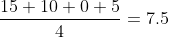Scan QR code or get instant email to install app

Question:

# Given the observations 45, 20, 30, and 25, the mean absolute deviation is closest to:

A 7.5.
explanation

1212The mean absolute deviation is the mean of the absolute values of the differences between each number and the mean. Because the mean is (45 + 20 + 30 + 25) I 4 = 30, the mean absolute deviation is